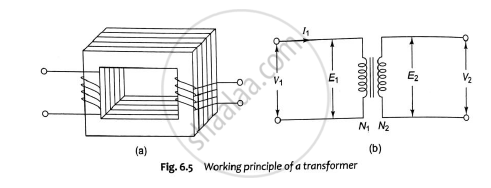# Describe the Basic Principle of Operation of a Single Phase Transformer and Derive the Emf Equation. - Basic Electrical and Electronics Engineering

Sum

Describe the basic principle of operation of a single phase transformer and derive the emf equation.

#### Solution

When an alternating voltage 𝑉1 is applied to a primary winding, an alternating current 𝐼1flows in it producing an alternating flux in the core. As per Faraday’s laws of electromagnetic induction, an emf 𝑒1 is induced in the primary winding.e_1=-N_1(dvarphi)/(dt)

Where 𝑁1 is the number of turns in the primary winding. The induced emf in the primary winding is nearly equal and opposite to the applied voltage 𝑉1.
Assuming leakage flux to be negligible, almost the flux produced in primary winding links with the secondary winding. Hence, an emf 𝑒2 is induced in the secondary winding.

e_2=-N_2(dvarphi)/(dt)

Where 𝑁2 is the number of turns in the secondary winding. If the secondary circuit is closed through the load, a current 𝐼2 flows in he secondary winding. Thus energy is transferred from the primary winding to the secondary winding.

EMF EQUATION.

As the primary winding is excited by a sinusoidal alternating voltage, an alternating current flows in the winding producing a sinusoidally varying flux 𝜑 in the core.

𝜑=𝜑𝑚𝑠𝑖𝑛𝜔𝑡

As per Faraday’s law of electromagnetic induction an emf 𝑒1 is induced in the primary winding.

e_1=-N_1(dvarphi)/(dt)

e_1=-N_1(dvarphi)/(dt)(𝜑𝑚𝑠𝑖𝑛𝜔𝑡)

𝑒1=−𝑁1𝜑𝑚𝜔𝑐𝑜𝑠𝜔𝑡 = −𝑁1𝜑𝑚𝜔sin (𝜔𝑡−90°) = 2𝜋𝑓𝑁1𝜑𝑚𝜔sin (𝜔𝑡−90°)
Maximum value of induced emf = 2𝜋𝑓𝜑𝑚𝑁1
Hence, rms value of induced emf in primary winding is given by,

E_1=(E_(max))/sqrt2=(2pifN_1varphi_m)/sqrt2=4.44 fN_1varphi_m

Similarly rms value of induced emf in the secondary winding is given by,

𝐸2=4.44𝑓𝑁2𝜑𝑚

Also, E_1/N_1=E_2/N_2=4.44fvarphim

Thus emf per turn is same in primary and secondary winding and an equal emf is induced in each turn of the primary and secondary winding.

Concept: Working Principle of Single Phase Transformer
Is there an error in this question or solution?
2018-2019 (December) CBCGS

Share